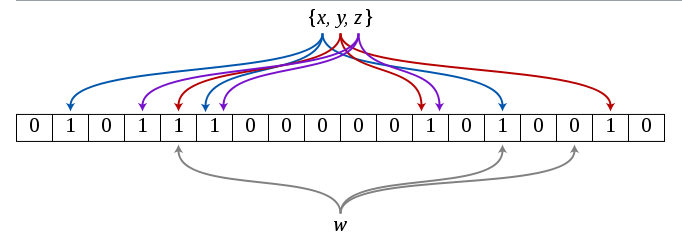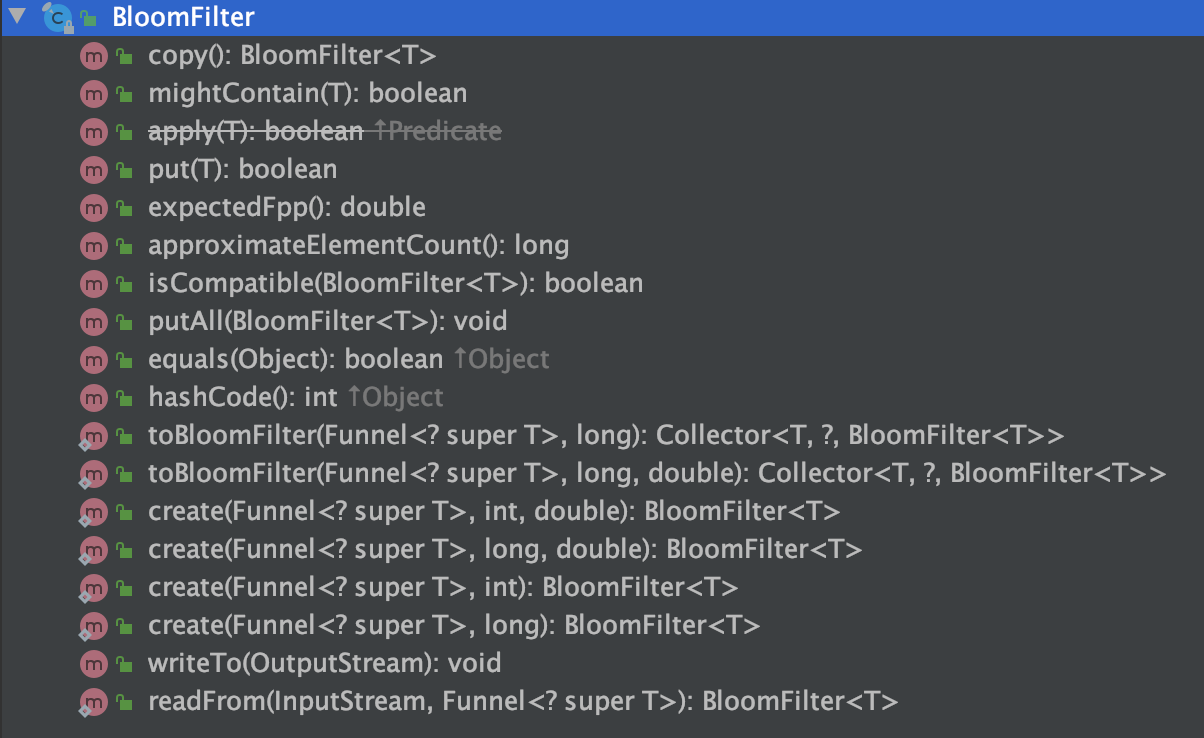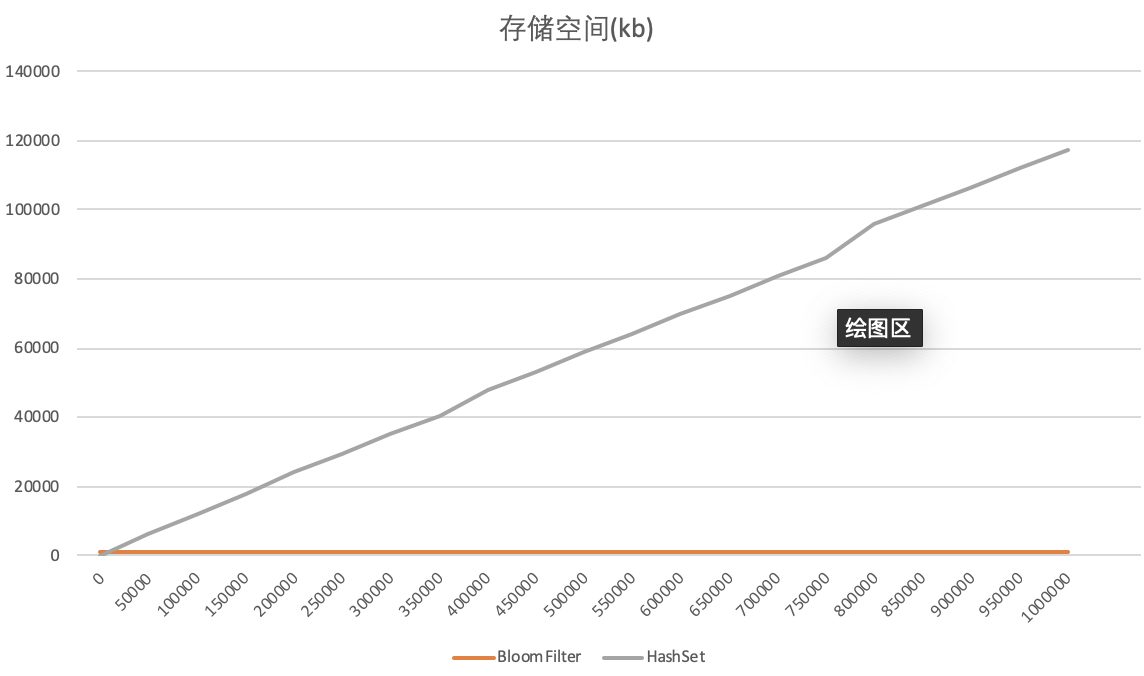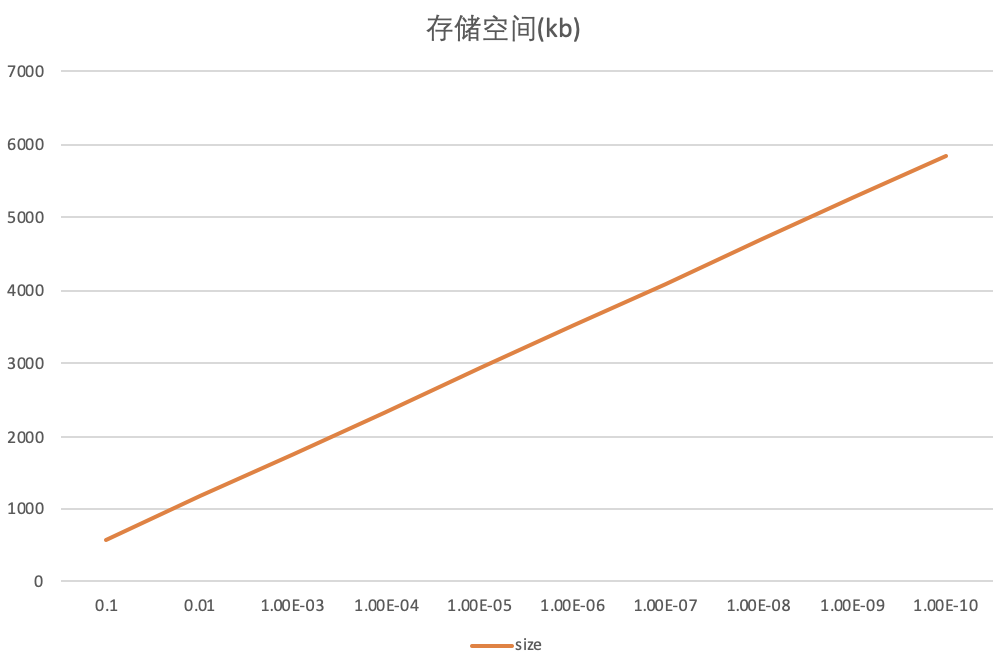# 布隆过滤器(BloomFilter)原理 实现和性能测试

## BloomFilter的原理

Bitmap的局限在于它的存储数据类型有限，只能存0-m之间的数，其他的数据就存不了了。如果我们想存字符串或者其他数据怎么办？其实也简单，只需要实现一个hash函数，将你要存的数据映射到0-m之间就行了。这里假设你的hash函数产生的映射值是均匀的，我们来计算下一个m位的Bitmap到底能存多少数据?

$$1 - \frac{1}{m}$$

$$(1 - \frac{1}{m})^n$$

$$P_1 = 1 - (1 - \frac{1}{m})^n$$

$$(1 - \frac{1}{m})^k$$

$$(1 - \frac{1}{m})^{kn}$$

$$1 - (1 - \frac{1}{m})^{kn}$$

$$\left(1-\left[1-\frac{1}{m}\right]^{k n}\right)^{k} < (1 - \left[1 - \frac{1}{m}\right]^n)$$

$$\left(1-\left[1-\frac{1}{m}\right]^{k n}\right)^{k} \approx\left(1-e^{-k n / m}\right)^{k}$$## 如何确定最优的m和k？

$$p \approx \left(1-e^{-k n / m}\right)^{k} \ (1)$$

$$k=\frac{m}{n} \ln2 \ (2)$$

$$p=\left(1-e^{-\left(\frac{m}{n} \ln 2\right) \frac{n}{m}}\right)^{\frac{m}{n} \ln 2} \ (3)$$

$$\ln p=-\frac{m}{n}(\ln 2)^{2}$$

$$m=-\frac{n \ln p}{(\ln 2)^{2}}$$

## guava中的BloomFilter

BloomFilter无法通过new去创建新对象，而它提供了create静态方法来生成对象，其核心方法如下。

  static <T> BloomFilter<T> create(
Funnel<? super T> funnel, long expectedInsertions, double fpp, Strategy strategy) {
checkNotNull(funnel);
checkArgument(
expectedInsertions >= 0, "Expected insertions (%s) must be >= 0", expectedInsertions);
checkArgument(fpp > 0.0, "False positive probability (%s) must be > 0.0", fpp);
checkArgument(fpp < 1.0, "False positive probability (%s) must be < 1.0", fpp);
checkNotNull(strategy);

if (expectedInsertions == 0) {
expectedInsertions = 1;
}

long numBits = optimalNumOfBits(expectedInsertions, fpp);
int numHashFunctions = optimalNumOfHashFunctions(expectedInsertions, numBits);
try {
return new BloomFilter<T>(new LockFreeBitArray(numBits), numHashFunctions, funnel, strategy);
} catch (IllegalArgumentException e) {
throw new IllegalArgumentException("Could not create BloomFilter of " + numBits + " bits", e);
}
}


- funnel 用来对参数做转化,方便生成hash值
- expectedInsertions 预期插入的数据量大小，也就是上文公式中的n
- fpp 误判率，也就是上文公式中的误判率p
- strategy 生成hash值的策略，guava中也提供了默认策略，一般不需要你自己重新实现

  static int optimalNumOfHashFunctions(long n, long m) {
// (m / n) * log(2), but avoid truncation due to division!
return Math.max(1, (int) Math.round((double) m / n * Math.log(2)));
}
static long optimalNumOfBits(long n, double p) {
if (p == 0) {
p = Double.MIN_VALUE;
}
return (long) (-n * Math.log(p) / (Math.log(2) * Math.log(2)));
}## 使用案例

• HBase、BigTable、Cassandra、PostgreSQ等著名开源项目都用BloomFilter来减少对磁盘的访问次数，提升性能。
• Chrome浏览器用BloomFilter来判别恶意网站。
• 爬虫用BloomFilter来判断某个url是否爬取过。
• 比特币也用到了BloomFilter来加速钱包信息的同步。
……

## 和HashSet对比

### 测试环境

guava(28.1)BloomFilter，JDK11(64位) HashSet

### 测试方式

#### BloomFilter vs HashSet#### 在不同fpp下的存储表现fpp,size
0.1,585.453125
0.01,1170.4765625
1.0E-3,1755.5
1.0E-4,2340.53125
1.0E-5,2925.5546875
1.0E-6,3510.578125
1.0E-7,4095.6015625
1.0E-8,4680.6328125
1.0E-9,5265.65625
1.0E-10,5850.6796875


## 参考资料

### 觉得文章有用就打赏一下文章作者

#### 支付宝扫一扫打赏#### 微信扫一扫打赏